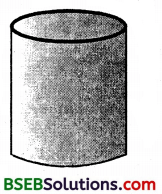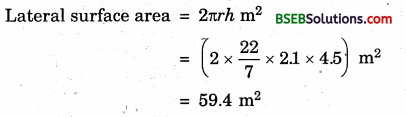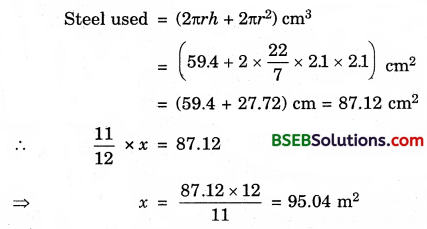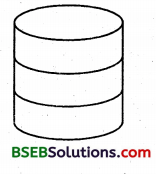# Bihar Board Class 9th Maths Solutions Chapter 13 Surface Areas and Volumes Ex 13.2

Bihar Board Class 9th Maths Solutions Chapter 13 Surface Areas and Volumes Ex 13.2 Textbook Questions and Answers.

## BSEB Bihar Board Class 9th Maths Solutions Chapter 13 Surface Areas and Volumes Ex 13.2Question 1.
The curved surface area of a right circular cylinder of height 14 cm is 88 cm². Find the diameter of the base of the cylinder.
Solution:
Let r be the radius of the base and h = 14 cm be the height of the cylinder. Then,
Curved surface area = 2πrhQuestion 2.
It is required to make a closed cylindrical tank of height 1 m and base diameter 140 cm from a metal sheet. How many square metres of the sheet are required for the same?
Solution:
Let r be the radius of the base and h be the height of the cylinder.
Metal sheet required to make a closed cylindrical tank = Its total surface areaHence, the sheet required = 7.48 m².Question 3.
A metal pipe is 77 cm long. The inner diameter of a cross-section is 4 cm, the outer diameter being 4.4 cm (see figure). Find its(i) inner curved surface area,
(ii) outer curved surface area,
(iii) total surface area.
Solution:
We have, R = external radius = $$\frac { 4.4 }{ 2 }$$ cm = 2.2 cm
r = internal radius = $$\frac { 4 }{ 2 }$$ cm = 2 cm
h = length of the pipe = 77 cm.
(i) Inner curved surface = 2πrh cm²
= 2 x $$\frac { 22 }{ 7 }$$ x 2 x 77 cm²
= 968 cm²

(ii) Outer curved surface = 2πRh cm²
= 2 x $$\frac { 22 }{ 7 }$$ x 2.2 x 77 cm²
= 1064.8 cm²

(iii) Total surface area of a pipe = Inner curved surface area + outer curved surface area + areas of two basesQuestion 4.
The diameter of a roller is 84 cm and its length is 120 cm. It takes 500 complete revolutions to move once over to level a playground. Find the area of the playground in m².
Solution:
The length of the roller is 120 cm i.e., h = 1.2 m and radius of the cylinder (i.e., roller) = $$\frac { 84 }{ 2 }$$ cm = 42 cm = 0.42 m.
Distance covered by roller in one revolution
= Its curved surface area
= 2πrh
= (2 x $$\frac { 22 }{ 7 }$$ x 0.42 x 1.2) m²
= 3.168 m²
Area of the playground = Distance covered by roller in 500 revolution
= (500 x 3.168) m²
= 1584 m²Question 5.
A cylindrical pillar is 50 cm in diameter and 3.5 m in height. Find the cost of painting the curved surface of the pillar at the rate of Rs 12.50 per m².
Solution:
Let r be the radius of the base and h be the height of the pillar.
∴ r = $$\frac { 50 }{ 2 }$$ cm = 25 cm = 25 m and h = 3.5 m.
Curved surface = 2πrh
= (2 x $$\frac { 22 }{ 7 }$$ x 0.25 x 3.5) m²
= 5.5 m²
Cost of painting the curved surface @ Rs 12.50 per m²

Question 6.
Curved surface area of a right circular cylinder is 4.4 m². If the radius of the base of the cylinder is 0. 7 m, find its height.
Solution:
Let r be the radius of the base and h be| the height of the cylinder. Then,
Curved surface area = 4.4 m²
⇒ 2πrh = 4.4
⇒ 2 x $$\frac { 22 }{ 7 }$$ x 0.7 x h = 4.4 [∵ r = 0.7 m]
⇒ h = $$\left(\frac{4.4 \times 7}{2 \times 22 \times 0.7}\right)$$ m = 1 m
Thus, the height of the cylinder = 1 metre.Question 7.
The inner diameter of a circular well is 3.5 m. It is 10 m deep. Find
(i) its inner curved surface area,
(ii) the cost of plastering this curved surface at the rate of Rs 40 per m².
Solution:
(i) Let r be the radius of the face and h be depth of the well. Then,
Curved surface = 2nrh
= (2 x $$\frac { 22 }{ 7 }$$ x $$\frac { 3.5 }{ 2 }$$ x 10) m² = 110 m²

(ii) Cost of plastering is Rs 40 per m²
∴Cost of plastering the curved surface = Rs (110 x 40)
= Rs 4400.

Question 8.
In a hot water heating system, there is a cylindrical pipe Pf length 28 m and diameter 5 cm. Find the total radiating surface in the system.
Solution:
Total radiating surface in the system
= Curved surface area of the pipe
= 2πrh,
where r = $$\frac { 5 }{ 2 }$$ cm = 2.5 cm = $$\frac { 2.5 }{ 100 }$$ m = 0.025 m and h = 28 m
= (2 x $$\frac { 22 }{ 7 }$$ x 0.025 x 28) m² = 4.4 m²Question 9.
Find (i) the lateral or curved surface.area of a cylindrical petrol storage tank that is 4.2 m in diameter and 4.5 m high.
(ii) how much steel was actually used, if $$\frac { 1 }{ 12 }$$ of the steel actually used was wasted in making the closed tank.
Solution:
(i) Here, r = ($$\frac { 4.2 }{ 2 }$$)m = 2.1 m and h = 4.5 m.(ii) Since $$\frac { 1 }{ 12 }$$ of the actual steel used was wasted, the area of the steel which has gone into the tank = (1 – $$\frac { 1 }{ 12 }$$) of x = $$\frac { 11 }{ 12 }$$ of x.Hence, the actual area of the steel used = 95.04 m²Question 10.
In figure, you see the frame of a lampshade. It is to be covered with a decorative cloth. The frame has a base diameter of 20 cm and height of 30 cm. A margin of 2.5 cm is to be given for folding it over the top and bottom of the frame. Find how much cloth is required for covering the lampshade.Solution:
Here r = ($$\frac { 20 }{ 2 }$$) cm = 10 cm and h = 30 cm + 2 x 2.5 cm (i.e margin) = 35 cm.
Cloth required for covering the lampshade
= Its curved surface area
= 2πrh
= (2 x $$\frac { 22 }{ 7 }$$ x 10 x 35) cm²
= 2200 cm²

Question 11.
The students of a Vidyalaya were asked to participate in a competition for making and decorating penholders in the shape of a cylinder with a base, using cardboard. Each penholder was to be of radius 3 cm and height 10.5 cm. The Vidyalaya was to supply the competitors with cardboard. If there were 35 competitors, how much cardboard was required to be bought for the competition?
Solution:
Cardboard required by each competitor = Curved surface area of one penholder + base area
= 2πrh + πr² where r = 3 cm, h = 10.5 cm
= [(2 x $$\frac { 22 }{ 7 }$$ x 3 x 10.5) + $$\frac { 22 }{ 7 }$$ x 9] cm²
= (198 + 28.28) cm²
= 226.28 cm² (approx.)
Cardboard required for 35 competitors = (35 x 226.28) cm²
= 7920 cm² (approx.)